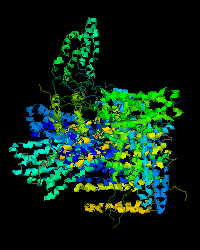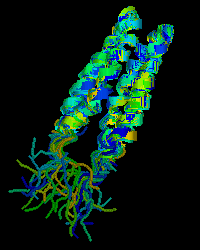# Protein Superposition using Biopython

For tasks like homology modelling, it is often useful to superimpose different (but related) protein structures on top of each other for comparison. BioPython's Bio.PDB module includes code to do all this...

When comparing two structures, you must have a mapping between equivalent amino acids. Then a distance measure can be used, such as the Root Mean Square Deviation (RMSD) between the Cα co-ordinates of these paired amino acids. A linear algebra trick called Singular Value Decomposition (SVD) is then used to find a rotation and translation which will minimise this distance.

On this page I'm going to discuss a special case - aligning alternative 3D structures of the same protein. In this situation, both structures have the same residues - so defining the mapping between atoms needed to calculate the distance is trivial.

When protein structures are solved by crystallography, there is usually a single solved structure. When NMR is used however, a range of possible models are generated. Some PDB files for NMR structures have the alternative structures provided pre-aligned. For others, this is not the case...

## An example PDB file

One NMR structure where the alternative models are not aligned is PDB structure 1JOY, the histidine kinase homo-dimeric domain of EnvZ from E. coli.

This PDB file contains twenty-one models of a dimer structure (identical chains A and B). Each model is apparently stored at a random orientation (shown below left). The following python script will do twenty pairwise alignments in order to superimpose the later models onto the first model - and output a new PDB with the new co-ordinates (shown below right).Before After

The above simple illustrations used OpenRasMol, showing the protein secondary structure using its "cartoon" representation, and coloured by chain.

It should be clear from the second image that in each model there is good agreement for the four alpha helices and their linking loops, but the free ends of the chains show a lot of variation which would spoil a superposition - they have been excluded when calculating the RMSD.

## The Python Script

As with most python scripts, this one starts by importing some libraries, and setting up some constants:

```import Bio.PDB
import numpy

pdb_code = "1JOY"
pdb_filename = "%s.pdb" % pdb_code
pdb_out_filename = "%s_aligned.pdb" % pdb_code

use_str = '-----------RTLLMAGVSHDLRTPLTRIRLATEMMSEQDGYLAESINKDI---------------'
use = [letter<>"-" for letter in use_str]
assert len(use) == len(seq_str)```

The interesting bit above sets up a list of booleans (variable use), one for each residue in the protein chain, which specifies wheather or not this residue should be included in the distance calculations.

Then the PDB file is read into the variable structure using Bio.PDB.PDBParser(). This provides a list of the 21 models in the PDB file. Using a for loop, each model is compared to the first model using Bio.PDB.Superimposer(), and its atoms locations updated.

```print "Loading PDB file %s" % pdb_filename
structure = Bio.PDB.PDBParser().get_structure(pdb_code, pdb_filename)

print "Everything aligned to first model..."
ref_model = structure
for alt_model in structure :
#Build paired lists of c-alpha atoms, ref_atoms and alt_atoms
#[code shown later]

#Align these paired atom lists:
super_imposer = Bio.PDB.Superimposer()
super_imposer.set_atoms(ref_atoms, alt_atoms)

if ref_model.id == alt_model.id :
#Check for self/self get zero RMS, zero translation
#and identity matrix for the rotation.
assert numpy.abs(super_imposer.rms) < 0.0000001
assert numpy.max(numpy.abs(super_imposer.rotran)) < 0.000001
assert numpy.max(numpy.abs(super_imposer.rotran) - numpy.identity(3)) < 0.000001
else :
#Update the structure by moving all the atoms in
#this model (not just the ones used for the alignment)
super_imposer.apply(alt_model.get_atoms())

print "RMS(first model, model %i) = %0.2f" % (alt_model.id, super_imposer.rms)```

The missing code above generates two matched lists of atoms for use by Bio.PDB.PDBParser(), and is shown below. It loops over each residue in each chain (doing the two models in parallel with the python zip() function):

```    ref_atoms = []
alt_atoms = []
for (ref_chain, alt_chain) in zip(ref_model, alt_model) :
for ref_res, alt_res, amino, allow in zip(ref_chain, alt_chain, seq_str, use) :
assert ref_res.resname == alt_res.resname
assert ref_res.id      == alt_res.id
assert amino == Bio.PDB.Polypeptide.three_to_one(ref_res.resname)
if allow :
#CA = alpha carbon
ref_atoms.append(ref_res['CA'])
alt_atoms.append(alt_res['CA'])```

In a general superposition problem, the matched residues may not be the same amino acid - so these assertions would have to be ommitted.

It would be trivial to extend this code to use all the backbone atoms (using their PDB names of 'N', 'C', 'CA', and 'C'). If you do have matched amino acids, you could even use every atom in each residue.

Notice that for this example both chain A and chain B have the same sequence, so the same list of allowed residues can be used for every chain - a slight simplification.

The final few lines of the script simply write the updated structure to a file.

```io=Bio.PDB.PDBIO()
io.set_structure(structure)
io.save(pdb_out_filename)```

Just for reference, these are the RMS distances calculated for 1JOY:

```RMS(first model, model 0) = 0.00
RMS(first model, model 1) = 1.37
RMS(first model, model 2) = 1.09
RMS(first model, model 3) = 1.15
RMS(first model, model 4) = 1.42
RMS(first model, model 5) = 1.29
RMS(first model, model 6) = 1.12
RMS(first model, model 7) = 1.10
RMS(first model, model 8) = 1.10
RMS(first model, model 9) = 1.50
RMS(first model, model 10) = 0.98
RMS(first model, model 11) = 1.05
RMS(first model, model 12) = 0.83
RMS(first model, model 13) = 1.42
RMS(first model, model 14) = 1.14
RMS(first model, model 15) = 1.21
RMS(first model, model 16) = 1.28
RMS(first model, model 17) = 1.10
RMS(first model, model 18) = 1.10
RMS(first model, model 19) = 1.50
RMS(first model, model 20) = 0.98```